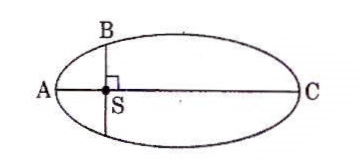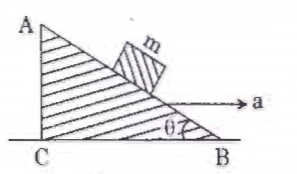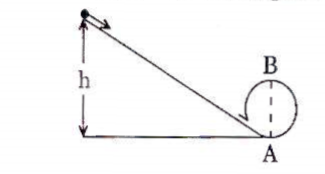# Q&A - Ask Doubts and Get Answers

Sort by :
Q

w

epididymis
Medical
1167 Views   |

. A sample of 0.1 g of water at 100°C and normal pressure (1.013 x 105 Nm-2) requires 54 cal of heat energy to convert to steam at 100°C. If the volume of the steam produced is 167.1 cc, the change in internal energy of the sample, is

• Option 1)

42.2 J

• Option 2)

208.7 J

• Option 3)

104.3 J

• Option 4)

84.5 J

As we have learned

First law of Thermodynamics -

Heat imported to a body in is in general used to increase internal energy and work done against external pressure.

- wherein

Option 1)

42.2 J

This is incorrect

Option 2)

208.7 J

This is correct

Option 3)

104.3 J

This is incorrect

Option 4)

84.5 J

This is incorrect

View More
Medical
92 Views   |

Two wires are made of the same material and have the same volume, The first wire has cross-sectional area A and the second wire has cross-sectional area 3A. If the length of the first wire is increased by  on applying a force F, how much force is needed to stretch the second wire by the same amount ?

• Option 1)

4 F

• Option 2)

6 F

• Option 3)

9 F

• Option 4)

F

As we have learned Young Modulus - Ratio of normal stress to longitudnal strain it denoted by Y - wherein F -  applied force A -  Area  -  Change in lenght l - original length       in second case ,    volume = constant  or              Option 1) 4 F This is incorrect Option 2) 6 F This is incorrect Option 3) 9 F This is correct Option 4) F This is incorrect
Medical
78 Views   |

The power radiated by a black body is P and it radiates maximum energy at wavelength, . If the temperature of the black body is now changed so that it radiates maximum energy at wavelength , the power

becomes nP. The value of n

• Option 1)

• Option 2)

• Option 3)

• Option 4)

As we have learned Radiant Power (P) - -       or n= 256/81     Option 1) This is correct Option 2) This is incorrect Option 3) This is incorrect Option 4) This is incorrect
Medical
645 Views   |

A small sphere of radius `r' falls from rest in a viscous liquid. As a result, heat is produced due to viscous force. The rate of production of heat when the sphere attains its terminal velocity, is proportional to

• Option 1)

r5

• Option 2)

r2

• Option 3)

r3

• Option 4)

r4

As we have learned Net force on the body -   - wherein    Rate of heat production   rate of heat            Option 1) r5 This is correct Option 2) r2 This is incorrect Option 3) r3 This is incorrect Option 4) r4 This is incorrect
Medical
930 Views   |

A solid sphere is in rolling motion. In rolling motion a body possesses translational kinetic energy (Kt) as well as rotational kinetic energy
(Kr) simultaneously. The ratio Kt : (Kt + Kr) for the sphere is

• Option 1)

10 : 7

• Option 2)

5 : 7

• Option 3)

7 : 10

• Option 4)

2 : 5

As we have learned Kinetic energy of a body in combined rotation and translation - - wherein =  Translational kinetic energy of centre of mass     =  Rotational kinetic energy about centre of mass             Option 1) 10 : 7 This is incorrect Option 2) 5 : 7 This is correct Option 3) 7 : 10 This is incorrect Option 4) 2 : 5 This is incorrect
Medical
205 Views   |

If the mass of the Sun were ten times smaller and the universal gravitational constant were ten times larger in magnitude, which of the following is not correct ?

• Option 1)

Time period of a simple pendulum on the Earth would decrease.

• Option 2)

Walking on the ground would become more difficult.

• Option 3)

Raindrops will fall faster.

• Option 4)

'g' on the Earth will not change.

As we have learned Acceleration due to gravity (g) - Force extended by earth on a body is gravity. Formula:    gravity density of earth Radius of earth   - wherein It's average value is on the surface of earth   For earth     rain drop will fall faster walking on the ground become more difficult  Time period of  simple pendulum will decrease           Option 1) Time period of a...
Medical
977 Views   |

The kinetic energies of a planet in an elliptical orbit about the Sun, at positions A, B and C are KA, KB and KC, respectively. AC is the major axis and SB is perpendicular to AC at the position of the Sun S as shown in the figure. Then• Option 1)

• Option 2)

• Option 3)

• Option 4)

As we have learned Kepler's 2nd law - Area of velocity =   Areal velocity   small area traced - wherein Simiar to Law of conservation of momentum  Angular momentum Known  as Law of Area           Option 1) This is incorrect Option 2) This is correct Option 3) This is incorrect Option 4) This is incorrect
Medical
588 Views   |

A solid sphere is rotating freely about its symmetry axis in free space. The radius of the sphere is increased keeping its mass same. Which of the following physical quantities would remain constant for the sphere ?

• Option 1)

Rotational kinetic energy

• Option 2)

Moment of inertia

• Option 3)

Angular velocity

• Option 4)

Angular momentum

As we have learned Rotational angular momentum -   - wherein = Moment of inertia about fixed axis of rotation = angular velocity of rotation      , Law of conservation of angular moment - - wherein If net torque is zero i.e. angular momentum is conserved only when external torque is zero .    when we have incraese radius of sphere keeping the mass same then moment of inertia...
Medical
78 Views   |

The moment of the force , at (2, 0 , -3 ) ,about the point (2, -2 , -2 ) is given by

• Option 1)

• Option 2)

• Option 3)

• Option 4)

As we have learned Torque -       - wherein This can be calculated by using either  = perpendicular distance from origin to the line of force. = component of force perpendicular to line joining force.                 Option 1) This is incorrect Option 2) This is incorrect Option 3) This is incorrect Option 4) This is correct
Medical
95 Views   |

A block of mass m is placed on a smooth inclined wedge ABC of inclination   as shown in the figure. The wedge is given an acceleration 'a' towards the right. The relation between a and  for the block to remain stationary on the wedge is• Option 1)

• Option 2)

• Option 3)

• Option 4)

4) a =gtan

Medical
319 Views   |

A toy car with charge q moves on a frictionless horizontal plane surface under the influence of a uniform electric field  . Due to the force q , its velocity increases from 0 to 6 m/s in one second duration. At that instant the direction of the field is reversed. The car continues to move for two more seconds under the influence of this field. The average velocity and the average speed of the toy car between 0 to 3 seconds are
respectively

• Option 1)

1 m/s, 3.5 m/s

• Option 2)

1 m/s , 3 m/s

• Option 3)

2 m/s , 4 m/s

• Option 4)

1.5 m/s , 3 m/s

As we have learned 2nd equation or Position- time equation - Displacement Initial velocity acceleration time   -    velocity increases from 0 to 6 m/s  velocity decreases from 6 to 0 m/s but car continues to move forword  since acceleration is same  car's velocity increases from 0 to -6 m/s  Distance travelled in first second Total displacement = 3m  Average velocity = 3/3 =1 m/s Total...
Medical
102 Views   |

Which one of the following statements is incorrect ?

• Option 1)

Frictional force opposes the relative motion.

• Option 2)

Limiting value of static friction is directly proportional to normal reaction.

• Option 3)

Rolling friction is smaller than sliding friction.

• Option 4)

Coefficient    of sliding friction    has
dimensions of length.

As we have learned Kinetic or Dynamic Friction -  kinetic friction   coefficient of kinetic friction R = reaction - wherein depends on the nature of surface in contact.    Coefficient of sliding friction is dimension lessThis is incorrect Option 1)  Frictional force opposes the relative motion. This is incorrect Option 2)  Limiting value of static friction is directly proportional to...
Medical
980 Views   |

A moving block having mass m, collides with another stationary block having mass 4m. The lighter block comes to rest after collision. When the initial velocity of the lighter block is v, then the value of coefficient of restitution (e) will be

• Option 1)

0.8

• Option 2)

0.25

• Option 3)

0.5

• Option 4)

0.4

As we have learned Coffecient of Restitution (e) - - wherein Ratio of relative velocity after collision to relative velocity before collision.    Let velocity of 4m mass is v after collision then  e=0.25       Option 1) 0.8 This is incorrect Option 2) 0.25 This is correct Option 3) 0.5 This is incorrect Option 4) 0.4 This is incorrect
Medical
79 Views   |

Three objects, A : (a solid sphere), B : (a thin circular disk) and C : (a circular ring), each have the same mass M and radius R. They all spin with the same angular speed  about their own symmetry axes. The amounts of work (W) required to bring them to rest, would satisfy the relation

• Option 1)

• Option 2)

• Option 3)

• Option 4)

As we have learned Kinetic energy of rotation - - wherein = moment of inertia about axis of rotation = angular velocity    work energy theorem states that work done =change in kinetic energy             Option 1) This is incorrect Option 2) This is incorrect Option 3) This is correct Option 4) This is incorrect
Medical
1434 Views   |

A body initially at rest and sliding along a frictionless track from a height h (as shown in the figure) just completes a vertical circle of diameter AB = D. The height h is equal to• Option 1)

• Option 2)

• Option 3)

• Option 4)

As we have learned If only conservative forces act on a system, total mechnical energy remains constant - -    For particles to complete circle  also          Option 1) This is incorrect Option 2) This is incorrect Option 3) This is incorrect Option 4) This is correct
Medical
94 Views   |

An inductor 20 mH, a capacitor 100 F and a resistor 50  are connected in series across a source of emf V = 10 sin 314 t. The power loss in circuit is

• Option 1)

2.74 W

• Option 2)

0.43 W

• Option 3)

0.79 W

• Option 4)

1.13 W

As we have learned Average power - -   power loss=            Option 1) 2.74 W This is incorrect Option 2) 0.43 W This is incorrect Option 3) 0.79 W This is correct Option 4) 1.13 W This is incorrect
Medical
441 Views   |

A thin diamagnetic rod is placed vertically between the poles of an electromagnet. When the current in the electromagnet is switched on, then the diamagnetic rod is pushed up, out of the horizontal magnetic field. Hence the rod gains gravitational potential energy. The work required to do this comes from

• Option 1)

the lattice structure of the material of the rod

• Option 2)

the magnetic field

• Option 3)

the current source

• Option 4)

the induced electric field due to the changing magnetic field

As we have learned Diamagnetic Substance - B<B0, B/B0<1 or  Relative permeability of diamagnetic substance. -   when current source is switched on, magnetic field sets up between poles on electromagnet Diamagnetic material due to its tendency to move from stronger to weaker field , is thus repelled out          Option 1) the lattice structure of the material of the rod This is...
Medical
918 Views   |

A metallic rod of mass per unit length 0.5 kg m-1 is lying horizontally on a smooth inclined plane which makes an angle of 30o with the horizontal. The rod is not allowed to slide down by flowing a current through it when a magnetic field of induction 0.25 T is acting on it in the vertical direction. The current flowing in the rod to keep it stationary is

• Option 1)

14.76 A

• Option 2)

5.98 A

• Option 3)

7.14 A

• Option 4)

11.32 A

As we have learned Force on a current carrying conductor - - wherein idl=current element    To keep the rod stationary  or  angle between rod and magnetic field is 90-  i.e    or          Option 1) 14.76 A This is incorrect Option 2) 5.98 A This is incorrect Option 3) 7.14 A This is incorrect Option 4) 11.32 A This is correct
Medical
258 Views   |

The electrostatic force between the metal plates of an isolated parallel plate capacitor C having a charge Q and area A, is

• Option 1)

proportional to the square root of the distance between the plates.

• Option 2)

linearly proportional to the distance between the plates.

• Option 3)

independent of the distance between the plates.

• Option 4)

inversely proportional to the distance between the plates.

As we have learned @7907 F is independent of distance between the plates Option 1)  proportional to the square root of the distance between the plates. Option 2)  linearly proportional to the distance between the plates. Option 3)  independent of the distance between the plates. Option 4) inversely proportional to the distance between the plates.
Exams
Articles
Questions